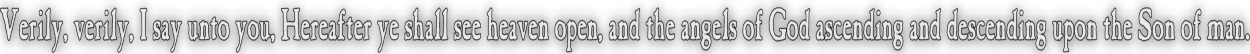None:
Polyps:
Strongs:MetaphysicsL(G) Implies Authority It is not true that L(G) allows HG(G) to be a set of beliefs held by another individual x in completeness. If we were to say HG(G) = HG(x) We would infer that "G", being perfect and able to define or refine virtues would exclude from x the property L(x) as if HG(G) were simply equal in every property to Hx(x). Then there can not be two such G with different virtues. L(G) would infer that the set of HG(G) \ L(G) is the maximum that may be attained by another individual x. It is true that if x were to hold every property in HG(G) then x would be for all intents and purposes, another 'G'. Therefore there is a distinct difference between the imperfect 'x' and the perfect 'G'. If x were to hold L(G) this would imply that 'G' be perfect and x defers to the choice of virtues made by 'G'. (Whereas 'G' is able to transform the trinity on virtue.) No such choice of transformation is able to be made by x. We say then that L(G) implies that 'G' is in authority to correct x on the basis that L(x) is less perfect than or "under" L(G). Then for a man x with HG(G) \ L(G) = Hx(x), x requires no correction on any fault, except to be "under" or subject to G. "x" is innocent, we may say, but without faith. We need to define some sense of "under". Clearly we can state that L(G) implies a correct set of virtues with a clearly distinct set of properties for "right and wrong". We can state that the principle L(G) is universal but only in order to differentiate 'G' from 'x' if the sets HG(G) \ L(G) and HG(x) are equal. We could state that the latter indicates there is no need to submit to L(G) if a man is "innocent" of any fault and has "almost" every virtue, (i.e. only without L(G) as faith) Rather we would consider that if HG(G) = HG(x) then since L(x) is also held, L(x) is "free" if L(x) is "under" L(G). That is, 'x' would never transform virtues in the trinity. It would be possible for a man to live without fault in a completely virtuous way under the authority of God. However if this is so, we need a clear distinction; a new operator that indicates that L(G) is "over" all L(x). Then we introduce Ay(x), which is taken to mean that y "has authority to correct" x if and only if y holds true to L(G). Then we merely state that AG(x) is universally true. We may equate AG(G) <=> L(G). (I.e. God is without fault.) Then we could state that Ax(G) may also be correct if x holds L(G) : We would assume that it is better for God to hold to His promises with His virtue intact then it is for Him to transform virtues, as if to avenge some fault, say. So the case HG(G) \ L(G) = HG(x) would indicate from AG(x) that HG(x) may be corrected to HG(G) if and only if Ax(G) is in agreement with AG(x). (i.e. both are simultaneously true.) Then it simply becomes a matter of agreement on right and wrong or on the specific set of virtues of God, without the need from x for a transformation of virtues by the trinity. Then the trinity may transform whenever we may put x=G. NB: we may substitute HG(G) for G in AG(x) as well as Ax(G), We may also even substitute HG(G) for G in HG(x) or Hx(G) also! This follows simply that every property in God is also understood in the understanding God has of Himself. More generally Hx(x) may be substituted for x in Hx(y) etc.. Continue To Next Page Return To Section Start Return To Previous Page'